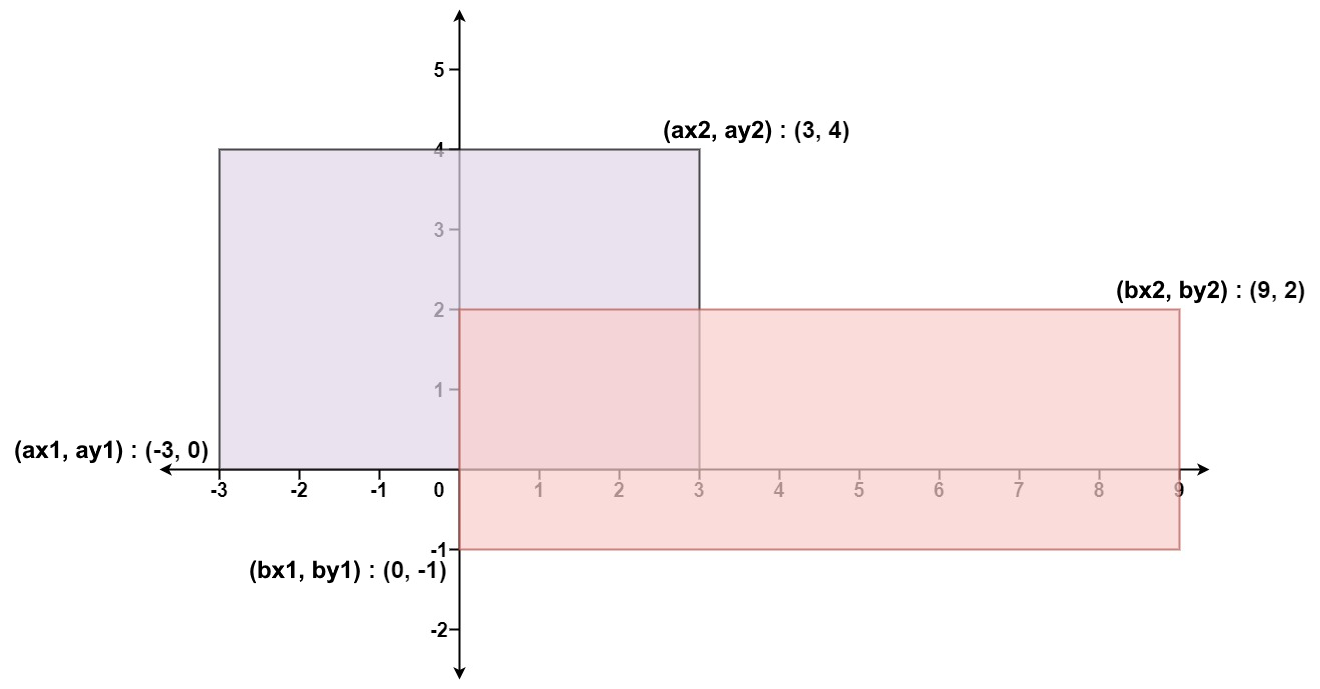# 2022-05-27

## ​231. Power of Two​

### Description

Given an integer `n`, return `true` if it is a power of two. Otherwise, return `false`.
An integer `n` is a power of two, if there exists an integer `x` such that `n == 2x`.
Example 1:
Input: n = 1
Output: true
Explanation: 20 = 1
Example 2:
Input: n = 16
Output: true
Explanation: 24 = 16
Example 3:
Input: n = 3
Output: false
Constraints:
• `-2^31 <= n <= 2^31 - 1`
Follow up: Could you solve it without loops/recursion?

### Solution

#### Approach #0

func isPowerOfTwo(n int) bool {
return n > 0 && n&(n-1) == 0
}

#### Approach #1

func isPowerOfTwo(n int) bool {
big := 1 << 30
return n > 0 && big%n == 0
}

## ​191. Number of 1 Bits​

### Description

Write a function that takes an unsigned integer and returns the number of '1' bits it has (also known as the Hamming weight).
Note:
• Note that in some languages, such as Java, there is no unsigned integer type. In this case, the input will be given as a signed integer type. It should not affect your implementation, as the integer's internal binary representation is the same, whether it is signed or unsigned.
• In Java, the compiler represents the signed integers using 2's complement notation. Therefore, in Example 3, the input represents the signed integer. `-3`.
Example 1:
Input: n = 00000000000000000000000000001011
Output: 3
Explanation: The input binary string 00000000000000000000000000001011 has a total of three '1' bits.
Example 2:
Input: n = 00000000000000000000000010000000
Output: 1
Explanation: The input binary string 00000000000000000000000010000000 has a total of one '1' bit.
Example 3:
Input: n = 11111111111111111111111111111101
Output: 31
Explanation: The input binary string 11111111111111111111111111111101 has a total of thirty one '1' bits.
Constraints:
• The input must be a binary string of length `32`.
Follow up: If this function is called many times, how would you optimize it?

### Solution

#### Approach #0

func hammingWeight(num uint32) int {
count := 0
for num > 0 {
num = num & (num - 1)
count++
}
return count
}

#### Approach #1

func hammingWeight(num uint32) int {
count := 0
for i := 0; i < 32; i++ {
if 1<<i&num > 0 {
count++
}
}
return count
}

## ​223. Rectangle Area​

### Description

Given the coordinates of two rectilinear rectangles in a 2D plane, return the total area covered by the two rectangles.
The first rectangle is defined by its bottom-left corner `(ax1, ay1)` and its top-right corner `(ax2, ay2)`.
The second rectangle is defined by its bottom-left corner `(bx1, by1)` and its top-right corner `(bx2, by2)`.
Example 1:Input: ax1 = -3, ay1 = 0, ax2 = 3, ay2 = 4, bx1 = 0, by1 = -1, bx2 = 9, by2 = 2
Output: 45
Example 2:
Input: ax1 = -2, ay1 = -2, ax2 = 2, ay2 = 2, bx1 = -2, by1 = -2, bx2 = 2, by2 = 2
Output: 16
Constraints:
• `-10^4 <= ax1, ay1, ax2, ay2, bx1, by1, bx2, by2 <= 10^4`

### Solution

#### Approach #0

func computeArea(ax1 int, ay1 int, ax2 int, ay2 int, bx1 int, by1 int, bx2 int, by2 int) int {
area1 := (ax2 - ax1) * (ay2 - ay1)
area2 := (bx2 - bx1) * (by2 - by1)
overlapWidth := min(ax2, bx2) - max(ax1, bx1)
overlapHeight := min(ay2, by2) - max(ay1, by1)
overlapArea := max(overlapWidth, 0) * max(overlapHeight, 0)
return area1 + area2 - overlapArea
}
func max(a, b int) int {
if a > b {
return a
}
return b
}
func min(a, b int) int {
if a < b {
return a
}
return b
}